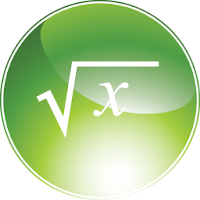# Math Formulary

3.0.26
2017-05-04
4.0 (3.3K)
Verified APK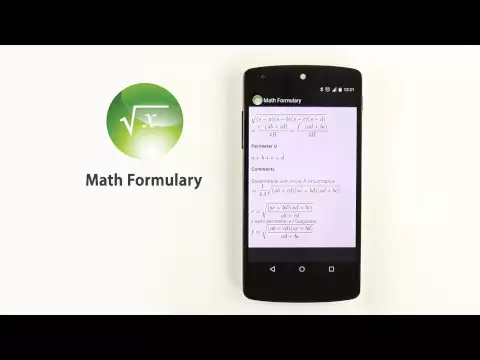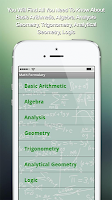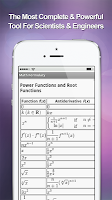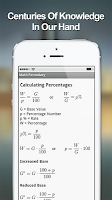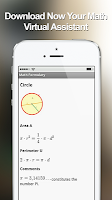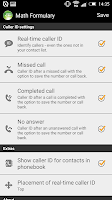##### Information
Name
Math Formulary
Version
3.0.26
File Size
6.99 MB
Updated on
May 4, 2017
750,000+
Requires
Android 2.3.4+ (Gingerbread MR1, API 10)
Category
Education
Developer
Sappalodapps
##### Description

One of the most powerful and incredible creations of the human mind, from Galileo to Einstein, is now available for you on your smartphone. Thanks to our app, Math Formulary, you'll have in your hands everything you need to handle every mathematical exercise or problem quickly and effectively.

This application is a complete math formulary, with all of math formulas needed from school to University. Thanks to our graphic design and software, the interface is very easy to use and intuitive. If you study or if you are a teacher, a scientist or a professional dealing with numbers and math formulas, you just discovered a powerful science tool, which will help you to improve a lot the quality and effectiveness of your work.

In the app you will find mathematical symbols explanation, free math worksheets, Algebra formulas, analysis formulas, geometry formulas, analytical geometry formulas, trigonometry formulas, logic formulas and basic arithmetic to help the younger students to learn this amazing and mysterious science.

★The app interface is organized in the following areas:
- Basic Arithmetic
- Algebra
- Analysis
- Geometry
- Trigonometry
- Analytical Geometry
- Logic

In every area, you will find the related math formulas and a complete explanation with graphics to help you understand the various topics. Math Formulary is a great tool for math and science teachers as well; all you need to have with you is your smartphone or tablet, no more books to carry during your work!